Wave Equation

Also found in: Dictionary, Thesaurus, Wikipedia.

Wave equation

The name given to certain partial differential equations in classical and quantum physics which relate the spatial and time dependence of physical functions. In this article the classical and quantum wave equations are discussed separately, with the classical equations first for historical reasons.

In classical physics the name wave equation is given to the linear, homogeneous partial differential equations which have the form of Eq. (1).

(1)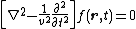Here &ugr; is a parameter with the dimensions of velocity; r represents the space coordinates x, y, z; t is the time; and ∇2 is Laplace's operator defined by
(2)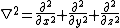Eq. (2). The function f( r ,t) is a physical observable; that is, it can be measured and consequently must be a real function.

The simplest example of a wave equation in classical physics is that governing the transverse motion of a string under tension and constrained to move in a plane.

A second type of classical physical situation in which the wave equation (1) supplies a mathematical description of the physical reality is the propagation of pressure waves in a fluid medium. Such waves are called acoustical waves, the propagation of sound being an example. A third example of a classical physical situation in which Eq. (1) gives a description of the phenomena is afforded by electromagnetic waves. In a region of space in which the charge and current densities are zero, Maxwell's equations for the photon lead to the wave equations (3).

(3)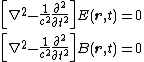Here E is the electric field strength and B is the magnetic flux density; they are both vectors in ordinary space. The parameter c is the speed of light in vacuum. See Electromagnetic radiation, Maxwell's equations

The nonrelativistic Schrödinger equation is an example of a quantum wave equation. Relativistic quantum-mechanical wave equations include the Schrödinger-Klein-Gordon equation and the Dirac equation. See Quantum mechanics, Relativistic quantum theory

McGraw-Hill Concise Encyclopedia of Physics. © 2002 by The McGraw-Hill Companies, Inc.
The following article is from The Great Soviet Encyclopedia (1979). It might be outdated or ideologically biased.

Wave Equation

a partial differential equation that describes the process of propagation of a disturbance in a medium. In the case of small disturbances and a homogeneous, isotropic medium, the wave equation has the form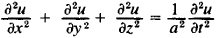where x, y, and z are spatial variables; t is time; u = u(x, y, z) is the function to be determined, which characterizes the disturbance at point (x, y, z) and time t; and a is the velocity of propagation of the disturbance. The wave equation is one of the fundamental equations of mathematical physics and is applied extensively. If u is a function of only two (one) spatial variables, then the wave equation is simplified and is called a two-dimensional (one-dimensional) equation. It permits a solution in the form of a“diverging spherical wave”:

u = f(t – r/a)/r

where f is an arbitrary function and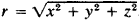. The so-called elementary solution (elementary wave) is of particular interest:

u = δ(t - r/a)/r

(where δ is the delta function); it gives the process of propagation for a disturbance produced by an instantaneous point source acting at the origin (when t = 0). Figuratively speaking, an elementary wave is an“infinite surge” on a circumference r = at that is moving away from the origin at a velocity a with gradually diminishing intensity. By superimposing elementary waves it is possible to describe the process of propagation of an arbitrary disturbance.

Small vibrations of a string are described by a one-dimensional wave equation: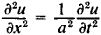In 1747, J. d’Alembert proposed a method of solving this wave equation in terms of superimposed forward and back waves: u = f(x - at) + g(x + at); and in 1748, L. Euler established that the functions f and g are determined by as-signing so-called initial conditions.

REFERENCE

Tikhonov, A. N., and A. A. Samarskii. Uravneniia matematicheskoi fiziki, 3rd ed. Moscow, 1966.

P. I. LIZORKIN

wave equation

[′wāv i‚kwā·zhən]
(physics)
In classical physics, a special equation governing waves that suffer no dissipative attenuation; it states that the second partial derivative with respect to time of the function characterizing the wave is equal to the square of the wave velocity times the Laplacian of this function. Also known as classical wave equation; d'Alembert's wave equation.
Any of several equations which relate the spatial and time dependence of a function characterizing some physical entity which can propagate as a wave, including quantum-wave equations for particles.
McGraw-Hill Dictionary of Scientific & Technical Terms, 6E, Copyright © 2003 by The McGraw-Hill Companies, Inc.
References in periodicals archive ?
Going back to Figure 1, we have compared the above results with those obtained by solving numerically the wave equation (1.5) with the same boundary data (see, e.g.,  or ).
In this paper, applying ideas from [16,18], we investigate the generalized Hyers-Ulam stability of the wave equation (3) with a source, where x > a and t > b with a,b [member of] R [union] {-[infinity]}.
Kuttler, "Propagation modeling over terrain using the parabolic wave equation," IEEE Transactions on Antennas and Propagation, vol.
In "CIP Method of Characteristics for the Solution of Tide Wave Equations," by Y.
By using tortoise coordinate transformation of the form [r.sub.*] = r + [(2[kappa]).sup.-1] ln(r - [r.sub.h]), the Klein-Gordon equation, the Maxwell's electromagnetic field equations, and the Dirac particles can be combined into a standard form of wave equation in a nonstationary or stationary spacetime (where k is the surface gravity).
and (10) to the standard wave equation for the elastic Euler beam
Based on the ideals and the techniques used in the mentioned papers, we studied a high-order asymptotic expansion of a weak solution for problem (1), where [(1).sub.1] has the form of a linear wave equation with nonlinear perturbations containing many small parameters [[epsilon].sub.1],...,[[epsilon].sub.p] as follows:
Numerical solution of regularized long wave equation by reduced differential transform method.
But compressibility and gravity are not factored in typical water wave equations that characterize ocean wave interactions, and "without compressibility and gravity, we cannot describe low-frequency sound waves correctly," says Kadri; therefore such equations normally don't apply to acoustic gravity waves.
This longitudinal mode displacement satisfies a wave equation for [epsilon], different from the transverse mode displacement wave equation for [[omega].sup.[mu]v].
which is derived by Fokas  in the process of extending the integrable Kadomtsev-Petviashvili (KP) and Davey-Stewartson (DS) equations to some new higher-dimensional nonlinear wave equations. In nonlinear wave theory, the KP equation and the DS equation are two important models, which have been proposed to describe the surface waves and internal waves in straits or channels of varying depth and width  and the evolution of a three-dimensional wave-packet on water of finite depth , respectively.

Site: Follow: Share:
Open / Close